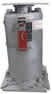# Variable Spring Supports vs Constant Spring Supports

##### By Dr. Hyder Husain Ph.D.Dec. 2, 2010

What is the difference between a variable & a constant spring support?
In a variable support, the force acting on the spring and hence the reactive force varies during the pipe travel, while the moment about the line of action is zero. In contrast, in a constant support, the fixed applied load remains uniform throughout its travel but the moment around a pivot point varies.

What is a variable support?
A variablesupport is essentially a spring, or series of springs, in a container. When the installed load “w” is applied, the spring is compressed through the distance W/k (where k is the spring rate) such that the reactive force exerted by the spring is also “w” under the equilibrium condition. As the pipe moves due to thermal expansion, it produces a deflection (∆L), causing a differential load (∆W=k ● ∆L), to act on the spring(s). Depending upon the direction of the movement, the change in load (∆W) will either add to or subtract from our installed load “w” to reach our final operating load (w1). In order to minimize the stress variations, the differential load (∆W) for a given variable spring support is limited to a maximum of 25% percent of the operating load (w1).

What is a constant support?
A constant support is a device comprised of a spring or series of springs and an integral cam mechanism. The external load of a constant support is fixed while its moment about the fixed pivot point varies during its travel (because the moment arm length changes).In order to maintain an equilibrium condition, the external force moment is balanced by the internal moment produced due to the spring’s compression or decompression about the pivot during the displacement of the pipe.

With an appropriate choice of moment arms, as developed by the cam geometry, and spring properties (i.e. spring rate), a resisting force can be provided that is nearly independent of position during its travel.

At each travel location of the applied load, the moment caused by the external load is balanced by the counter moment produced by the (compressed/decompressed) spring force with the appropriate moment arm. Typically, the variation of the active and reactive forces is very small (with a maximum deviation of 6%) and can be taken as a constant force while moving either upward or downward.

Top